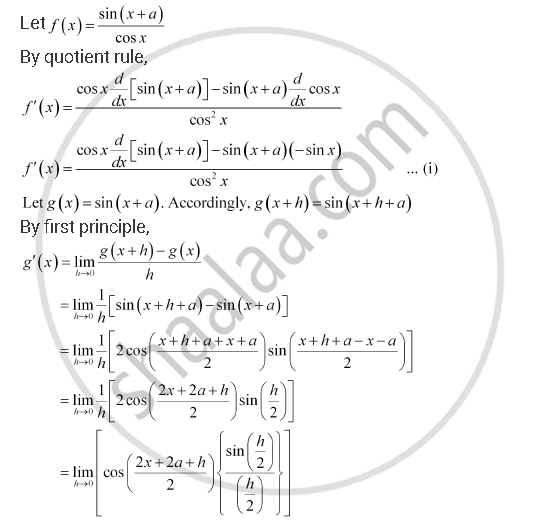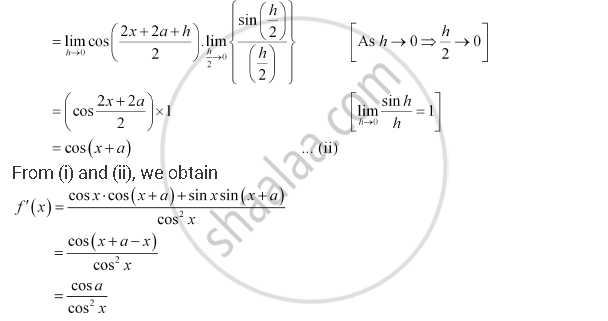Share

# Find the Derivative of the Following Functions (It is to Be Understood That A, B, C, D, P, Q, R And S Are Fixed Non-zero Constants And M And N Are Integers): (Sin(X + A))/ Cos X - Mathematics

#### Question

Find the derivative of the following functions (it is to be understood that abcdp, q, r and s are fixed non-zero constants and m and n are integers): (sin(x + a))/ cos x

#### SolutionIs there an error in this question or solution?

#### APPEARS IN

NCERT Solution for Mathematics Textbook for Class 11 (2018 (Latest))
Chapter 13: Limits and Derivatives
Q: 21 | Page no. 318

#### Video TutorialsVIEW ALL 

Solution Find the Derivative of the Following Functions (It is to Be Understood That A, B, C, D, P, Q, R And S Are Fixed Non-zero Constants And M And N Are Integers): (Sin(X + A))/ Cos X Concept: Derivative - Algebra of Derivative of Functions.
S